Examples of Predicate Logic Formulas

Only dogs bark.

Rephrase: It barks only if it is a dog.

Or equivalently: If it barks, then it is a dog.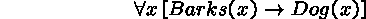Everyone has a father.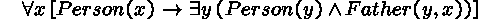Nobody is infallible.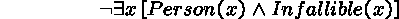Implicit Quantification

Sometimes the formal representation of a statement requires quantifiers, even though none of the telltale words ``all'', ``some'', etc. is present.

For example,

If a number is an integer, then it is a rational number
looks like a conditional statement, but is more accurately formalized as a universal statement,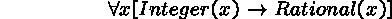Rephrasing the informal statement makes the use of a quantifier explicit.

Every integer is a rational number

Existential quantification can also be implicit.

The number 24 can be written as a sum of two even integers.Domains and Predicates

There are different ways of specifying the domain of a predicate variable.

(1) Explicitly indicate the domain: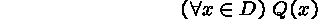(2) Represent the domain by a predicate: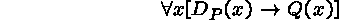whereis meant to be true if, and only if, x is an element of D.

A statement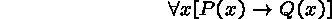is said to be vacuously true or true by default if P(x) is false for every x.

This implies that a statementis true whenever the domain D is empty, which reminds me of a tale (perhaps apocryphal) about the mathematician who proved all these wonderful properties about a special class of groups, of which it was ultimately proven that no groups were in this class ...

Multiple Quantifiers

Quantifiers can be nested, with alternations between universal and existential quantifiers.

Everybody loves somebody.
Somebody loves everybody.
These statements have similar structure, but with different order of quantifiers.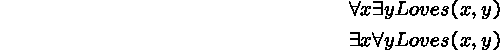Such statements are often difficult to evaluate.

Are the two statements equivalent?

Does one of them imply the other?

Consider two similar statements,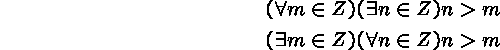where Z denotes the domain of integers.

Limits and Nested Quantifiers

Informally, a number L is the limit of a sequence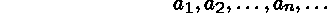if the valuesbecome arbitrarily close to L as n gets larger.

This concept can be formally defined in predicate logic as follows.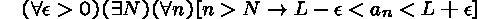The logical complexity of this formula (two alternations of quantifiers) explains why most students find it hard to understand the concept of a limit.

Note that a formula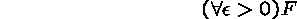is just a shorthand forEvaluation of Complex Formulas

The truth value of complex formulas with quantifiers may be difficult to determine.

Every even number is the sum of two primes.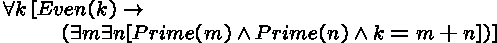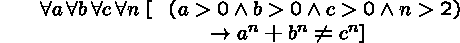The difficulty with evaluation of quantified statements, and a key difference between predicate and propositional logic, is that variables denote elements of some domain, which may be infinite.

For instance, a universal statement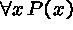is true if P(x) is true for all possible values of x.

If the given domain is infinite, e.g., the set of the integers or the real numbers, there are infinitely many cases to consider!

Angels and Devils

Sometimes it's helpful to pit an angel, whose job it is to make a formula true, against a devil, who attempts to make a formula false.

The two opponents scan a given formula, the angel making a move on an existential quantifier, whereas the devil takes his turn on a universal quantifier.

Each move consists of choosing a value for the quantified variable in question, dropping the quantifier, and applying the chosen substitution to the remaining formula.

Example.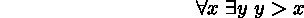1. Faced with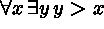, the devil chooses x=1,000.
2. The angel gets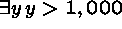and chooses y=1,001.
3. The game ends with the proposition 1,001 > 1,000, which is true. The angel wins.

The angel wins whenever the final proposition is true. Otherwise the devil wins.

More Challenging Game

Every integer has a (integer) square root.1. The devil begins and chooses x=4.
2. The angel gets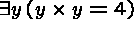and chooses y=2
3. The result is a true proposition,. The angel wins.
Poor strategy on the devil's part! Another try:

1. The devil begins by choosing x=3.
2. The angel gets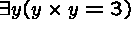and chooses y=1.
3. The result is a false proposition,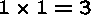. The devil wins.
This is a winning strategy for the devil! The angel has no chance (in this case).

It's All Strategy

If there is a winning strategy for the devil, the original formula is false.We have seen a winning strategy for the devil: choose x=3 (or x=5, or x=19, etc).

If there is a winning strategy for the angel, the original formula is true.A winning strategy for the angel is to choose the number n+1 for y, whenever the devil has initially chosen n for x.

Logical Equivalence and Consequence

Some relationships hold between formulas just on the basis of their syntactical form, regardless of the meaning of the predicates.

Examples are more generalized forms of the logical equivalences from propositional logic.That is, if two propositional formulas are logically equivalent, one may replace propositional formulas by arbitrary predicate logic formulas: the resulting formulas are still equivalent.

Other equivalences derive from more subtle connections between quantifiers and propositional connectives.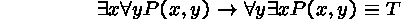Negations of Quantified Statements

The negation of a universal statement is logically equivalent to an existential statement: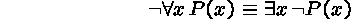Not all students drink.
Some student does not drink.

The negation of an existential statement is logically equivalent to a universal statement:No student failed the course.
All students passed the course.

The above equivalences indicate that only one of the two quantifiers is really needed.

Recall that a logical argument is a sequence of statements, of which the last one is called the conclusion, while all the others are called hypotheses.

An argument is valid if the conclusion is a logical consequence of the (conjunction of all the) hypotheses.

Example.

(1) Everyone is afraid of Dracula.
(2) Dracula is afraid only of me.
Therefore I am Dracula.

Is this argument valid?